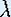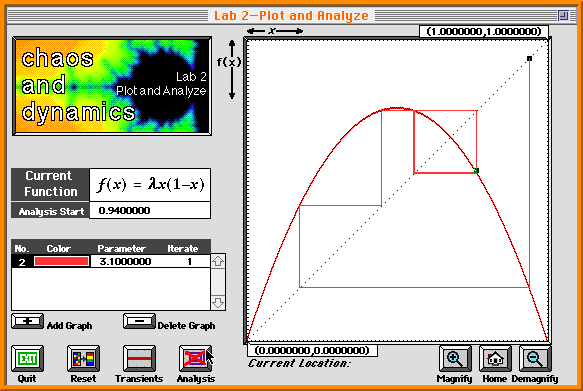Up: Iteration

# One-Dimensional Dynamical Systems

## Part 3: Iteration

#### Instructions for the Macintosh: Iterations

The software Chaos and Dynamics can be found in the apple-menu, under 'Math Apps'. Go to 'Devaney's Chaos Lab' and load 'Lab 1-Function Iteration'. When you load the Lab, the current function is automatically set to the Logistic map.Function Iteration with= 2.

#### Instructions to Iterate

• Push the Chart button to get a setup for plotting iterates against (discrete) time.

• Changeto 2: Notice the number marked Parameter under the Current Function box.

• You may wish to change the starting value x0 on top of the plot. (x0 should be chosen in the closed interval [0,1].) Then push Iterate.

• If you push Chart again the picture clears and you can compute another orbit. Experiment with different values forand x0.

#### Instructions for the Macintosh: Graphical Iteration

The software Chaos and Dynamics can be found in the apple-menu, under 'Math Apps'. Go to 'Devaney's Chaos Lab' and load 'Lab 2-Plot and Analyze'. For the Logistic map, pick avalue between 0 and 4, and iterate as follows:Visualizing an orbit with graphical iteration for the Logistic map.

#### Instructions to Iterate

• Push the Add Graph button, marked with a '+'. You should see a graph of the Logistic family.

• Change the parameter: Notice the number under the column marked Parameter. This is the value of. Edit this number (and hit return).

• Double click on any point in the graph window; the iteration will start with the x value of this point. This x value also appears in the Analysis Start box.

Alternatively, edit the Analysis Start box to the desired initial x value (and hit return). Then press Analysis.

• Press Analysis to stop the iteration, and try another point.

Up: IterationThe Geometry Center Home Page

Written by Hinke Osinga